# number of months left in the model calendarHi,

I'm looking for a way to reference the last month in the model calendar.

For Example My model Calendar is 1 previous year and 2 future years. Currently the last Period is June 2022 however when the model calendar increases i want the formula to reference the new end period.

I then want to count the months from the current period (item(time) to the End period (e.g. June 2022)

Ultimately my goal is to reference the number of months left in the time dimension.

Tagged:

•Step-1 Determine Start and End Times of Calender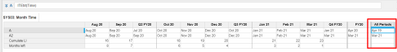Step-2: Obtain Start Period and End period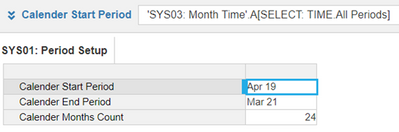Step-3: Calculate no. of months in calender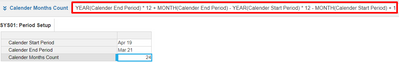Step-4: Cumulate LI to count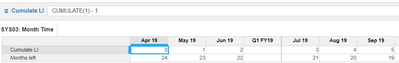Step-5: Final formula to subract the Cumulate LI to determine no. of months left.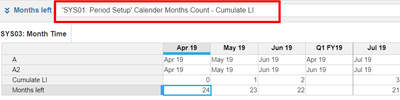Hope this helps!

•Hope this helps.

Months -> Basically shows all time periods of the model calendar

Final Month -> To get this month u need to select total of all time periods in Time settings

No of Months left -> Formula to get difference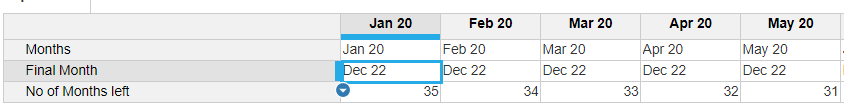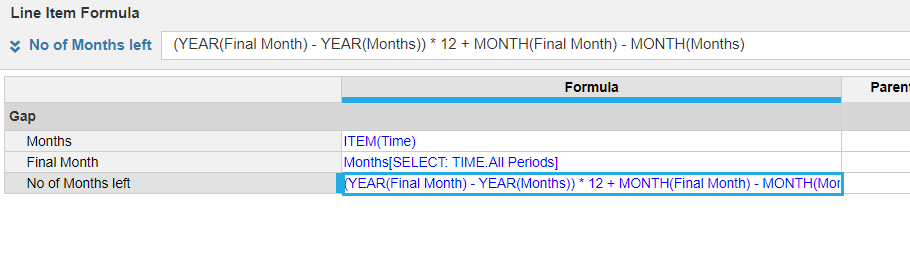Thanks & Regards,

•This can be achieved by using a combination of time system modules.

Firstly, ensure you have 'Total of All Time Periods,' selected in the model time settings.

Secondly, create a time properties module dimensioned only by time and set this to monthly time periods.

Create a line item called cumulate and enter the following formula =cumulate(1)

This will create a rolling total of all periods in the model calendar.

Create a second line item called Total number of periods and enter the following formula =cumulate[select:time.all periods] This will return the total of all time periods and hence the full number of periods in the model calendar.

Create a third line item called remaining periods and enter the following formula = Total number of periods - cumulate

This will return the difference between the each month and the total number of periods in the calendar.

You can then look against the month in this system model against the third line item.

•Simple and useful. thanks Sai

Regards

Rahul